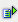The function counta (range) calculates the number of not empty cells within a range.

Syntax: =counta(range)Example

Cell A5 = counta(A1:A4)

 R / C A 1 1 2 text 3 4 3 5 3

The number of not empty cells in the range A1 to A4 is 3